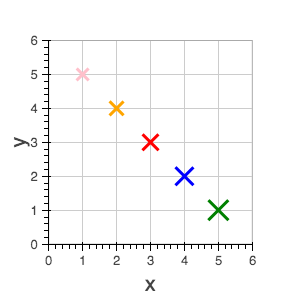BokehJS Interface Reference¶

Data Sources¶

Data is typically passed to bokehjs through a ColumnDataSource which has named columns of data that are available to glyph renderers. Note that all the columns in a given ColumnDataSource should have the same length. It is possible and often necessary to have different data sources for different renderers.

Warning

JavaScript null values are not valid inputs to BokehJS renderers. Please be aware that they will be silently interpreted as zero.

Line Properties¶

BokehJS exposes all the standard HTML5 canvas path stroking properties. All of these properties may take single values, or references to vectors of data. For instance, it is possible to draw multiple rectangles at once using the rect glyph. They may all be given the same line_width or each rectangle may be given its own line_width.

line_color
color to use to stroke lines with
line_width
line stroke width in units of pixels
line_alpha
floating point between 0 (transparent) and 1 (opaque)
line_join

how path segments should be joined together

line_cap

how path segments should be terminated

line_dash
array of integer pixel distances that describe the on-off pattern of dashing to use
line_dash_offset
the distance in pixels into the line_dash that the pattern should start from

Fill Properties¶

BokehJS exposes all the standard HTML5 path filling properties. All of these properties may take single values, or references to vectors of data. For instance, it is possible to draw multiple rectangles at once using the rect glyph. They may all be given the same fill_width, or each rectangle may be given its own fill_width.

fill_color
color to use to fill paths with
fill_alpha
floating point between 0 (transparent) and 1 (opaque)

Text Properties¶

BokehJS exposes most of the standard HTML5 text properties. All of these properties may take single values, or references to vectors of data. For instance, it is possible to draw multiple text items at once using the text glyph. They may all be given the same font_size or each line of text may be given its own font_size.

text_font
font name, e.g., 'times', 'helvetica'
text_font_size
font size in px, em, or pt, e.g., '12pt', '1.5em'
text_font_style

font style to use

• 'normal' normal text
• 'italic' italic text
• 'bold' bold text
text_color
color to use to render text with
text_alpha
floating point between 0 (transparent) and 1 (opaque)
text_align
horizontal anchor point for text: 'left', 'right', 'center'
text_baseline

vertical anchor point for text

• 'top'
• 'middle'
• 'bottom'
• 'alphabetic'
• 'hanging'

Note

There is currently only support for filling text. An interface to stroke the outlines of text has not yet been exposed.

Markers¶

Markers are a subset of BokehJS glyphs that have a uniform interface and are suitable for simple scatter type plots. All markers expose the following fields:

• x, y - center point coordinates
• size - screen units

and have Line Properties and Fill Properties as appropriate.

Glyphs¶

Bokeh plots are centered around glyphs, which generally have some combination of line, fill, or text properties, depending on what is appropriate for a given glyph. For example, the Circle glyph has both line and fill properties, but the Bezier glyph only has line properties.

Note

Each of the glyph images below links to a live JSFiddle.

annular_wedge¶

The annular_wedge glyph displays annular wedges centered at the given coordinates with the corresponding start_radius, end_radius, start_angle and end_angle.

Note

the direction field may be used to indicate which direction the drawing should occur between start_radius and end_radius.

• x, y - center point coordinates
• start_angle
• end_angle
• direction
• values: 'clock', 'anticlock'
• default: 'anticlock'
• Line Properties
• Fill Properties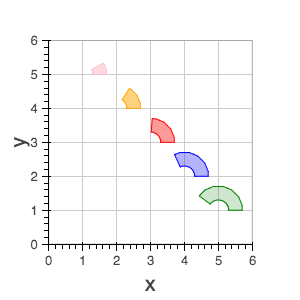annulus¶

The annulus glyph displays annular rings centered at the given coordinates with the corresponding start_radius and end_radius.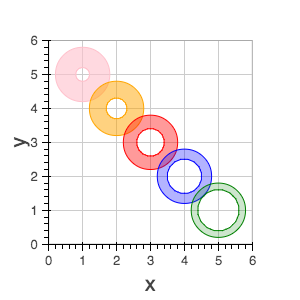arc¶

The annulus glyph displays circular line arcs centered at the given coordinates with the corresponding radius, start_angle and end_angle.

Note

the direction field may be used to indicate which direction the drawing should occur between start_radius and end_radius.

• x, y - center point coordinates
• start_angle
• end_angle
• direction
• values: ['clock' or 'anticlock']
• default: 'anticlock'
• Line Properties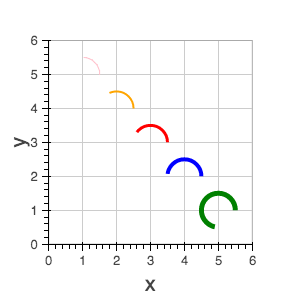asterisk¶

The asterisk glyph is a marker that displays asterisks at the given coordinates.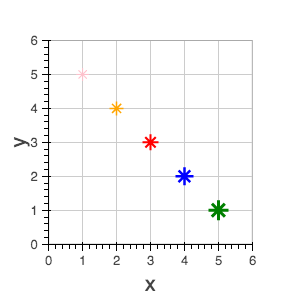bezier¶

The bezier glyph displays Bezier curves with the given starting, ending, and control points.

• x0, y0 - starting point coordinates
• x1, y1 - ending point coordinates
• cx0, cy0 - first control point coordinates
• cx1, cy1 - second control point coordinates
• Line Properties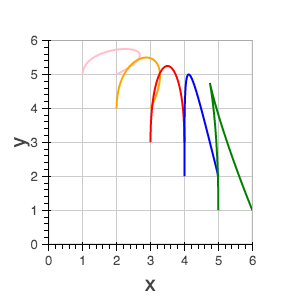circle¶

The circle glyph has two forms, a marker form that takes a size field or a non-marker form that takes a radius field.

 x, y - center point coordinates size Line Properties Fill Properties x, y - center point coordinates radius Line Properties Fill Properties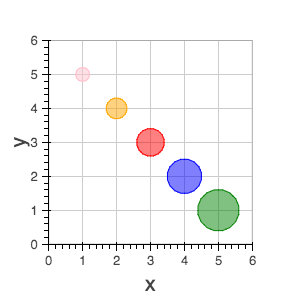circle_cross¶

The circle_cross glyph is a marker that displays circles together with a crossbar (+) at the given coordinates.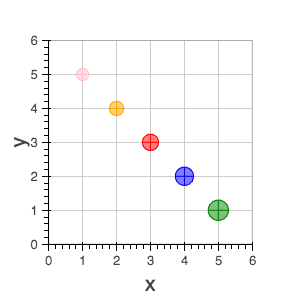circle_x¶

The circle_x glyph is a marker that displays circles together with an X at the given coordinates.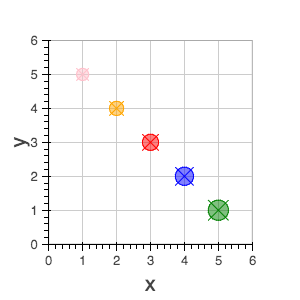cross¶

The cross glyph is a marker that displays crossbar symbols (+) at the given coordinates.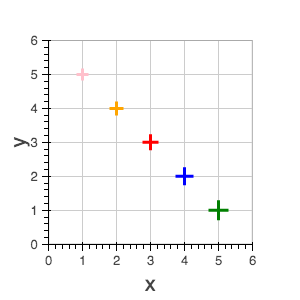diamond¶

The diamond glyph is a marker that displays diamonds at the given coordinates.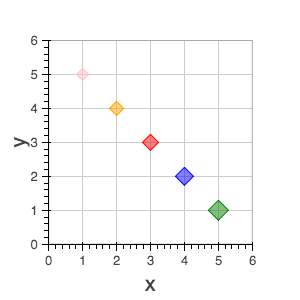diamond_cross¶

The diamond_cross glyph is a marker that displays diamonds together with a crossbar (+) at the given coordinates.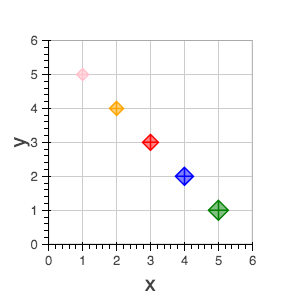image¶

The image glyph has two forms. The first form takes each image as a one-dimensional array of scalar values together with rows and cols fields that describe the two-dimensional shape of the array. The second form takes each image as a”array of arrays” (assumed to be non-ragged) and the shape is inferred automatically. A palette (string name of a built-in palette, currently) must also be supplied to use for color-mapping the scalar image.

Note

The image glyph is vectorized like other glyphs, i.e. it may be used to display several images at once.

Warning

The second (2D) form is significantly less efficient. It is currently used by the python interface to send data to the browser, but may be deprecated in the future.

 image - 1D array of data rows - number of rows in image cols - number of columns in image x, y - lower left dw - width on screen dh- height on screen palette image - 2D array of data x, y - lower left dw - width on screen dh- height on screen palette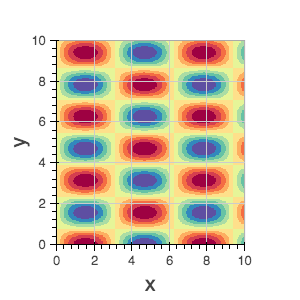image_rgba¶

The image_rgba glyph has two forms. The first form takes each image as a one-dimensional array of RGBA values (encoded as 32-bit integers) together with rows and cols fields that describe the two-dimensional shape of the array. The second form takes each image as a “array of arrays” (assumed to be non-ragged) and the shape is inferred automatically.

Note

The image_rgba glyph is vectorized like other glyphs, i.e. it may be used to display several images at once.

Warning

The second (2D) form is significantly less efficient. It is currently used by the python interface to send data to the browser, but may be deprecated in the future.

 image - 1D array of RGBA rows - number of rows in image cols - number of columns in image x, y - lower left dw - width on screen dh- height on screen image - 2D array of RGBA x, y - lower left dw - width on screen dh- height on screen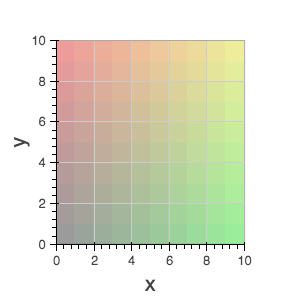image_url¶

The image_url glyph accepts the URLs of an images to display. The images are centered on the given coordinates and rotated by the given angles.

• x, y - center point coordinates
• url
• angle

inverted_triangle¶

The inverted_triangle glyph is a marker that displays upside-down triangles at the given coordinates.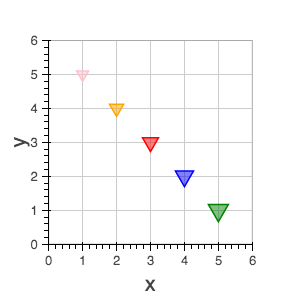line¶

The line glyphs displays a single line that connects several points given by the arrays of coordinates x and y.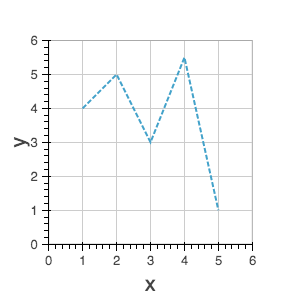multi_line¶

The multi_line glyphs displays several lines, each with points given by the arrays of coordinates that are the elements of xs and ys. This glyph is especially useful for implementing parallel coordinates plots, or plotting several aligned series simultaneously.

Note

For this glyph, the vector data is not simply an array of scalars, it is really an “array of arrays”.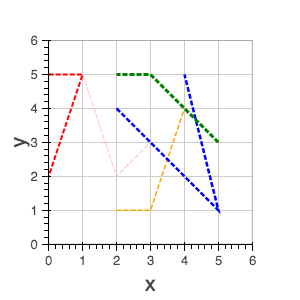oval¶

The oval glyph displays ovals centered on the given coordinates with the given dimensions and angle.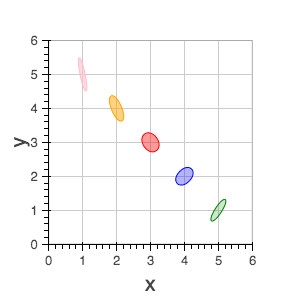patch¶

The line glyphs displays a single polygonal patch that connects several points given by the arrays of coordinates x and y.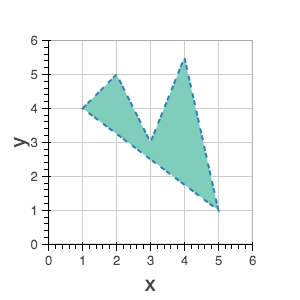patches¶

The patches glyphs displays several patches, each with points given by the arrays of coordinates that are the elements of xs and ys. This glyph is especially useful for implementing stacked area charts and cartograms.

Note

For this glyph, the vector data is not simply an array of scalars, it is really an “array of arrays”.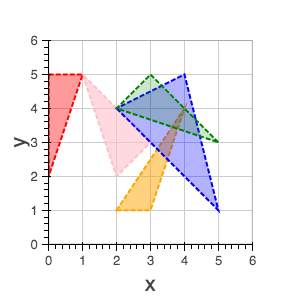The quad glyph displays axis-aligned rectangles with the given dimensions.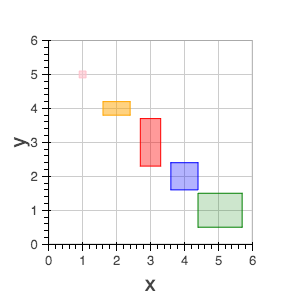The quadratic glyph displays quadratic curves with the given starting, ending, and control points.

• x0, y0 - starting point coordinates
• x1, y1 - ending point coordinates
• cx, cy - control point coordinates
• Line Properties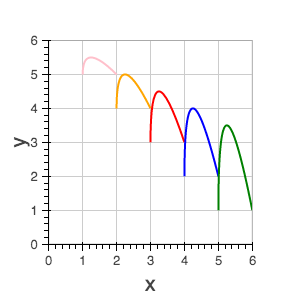ray¶

The ray glyph displays line segments starting at the given coordinate and extending the given length at the given angle.

• x0, y0 - starting point coordinates
• length - screen units
• angle
• default: 0
• Line Properties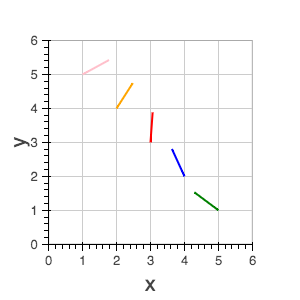rect¶

The rect glyph displays rectangles centered on the given coordinates with the given dimensions and angle.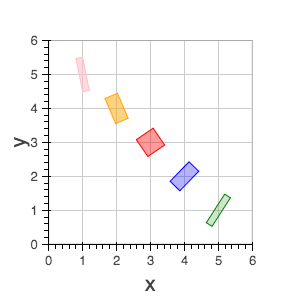segment¶

The segment glyph displays line segments with the given starting and ending coordinates.

• x0, y0 - starting point coordinates
• x1, y1 - ending point coordinates
• Line Properties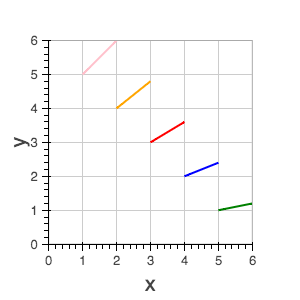square¶

The square glyph is a marker that displays squares at the given coordinates.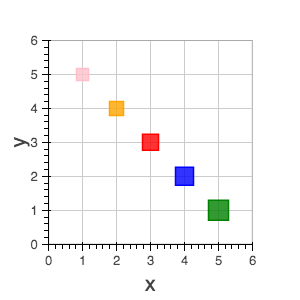square_cross¶

The square_cross glyph is a marker that displays squares together with a crossbar (+) at the given coordinates.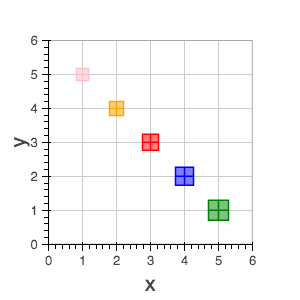square_x¶

The square_x glyph is a marker that displays squares together with an X at the given coordinates.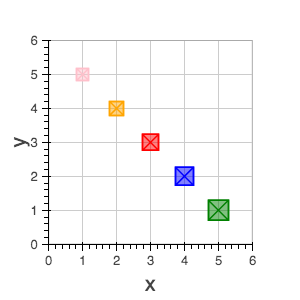text¶

The text glyph displays text at the given coordinates rotated by the given angle. The location of the coordinates relative to the text is indicated by the text properties.

• x, y - text coordinates (positioning determined by text properties)
• text
• angle
• default: 0
• Text Properties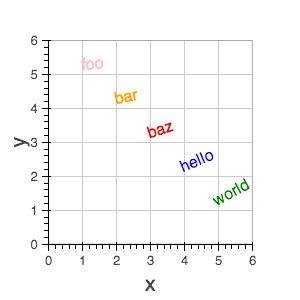triangle¶

The triangle glyph is a marker that displays triangles at the given coordinates.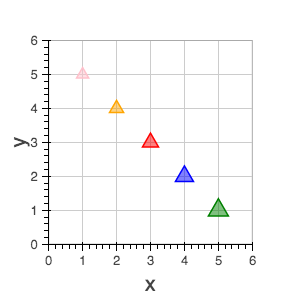wedge¶

The annular_wedge glyph displays circular wedges centered at the given coordinates with the corresponding radius, start_angle and end_angle.

Note

the direction field may be used to indicate which direction the drawing should occur between start_radius and end_radius.

• x, y - center point coordinates
• start_angle
• end_angle
• direction
• values: ['clock' or 'anticlock']
• default: 'anticlock'
• Line Properties
• Fill Properties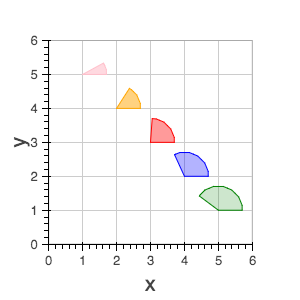x¶

The x glyph is a marker that displays X symbols at the given coordinates.